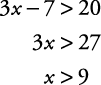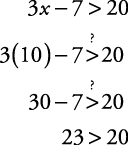## Linear Inequalities

inequality is a sentence using a symbol other than the equals sign (=). The most common inequality symbols are <, ≤, >, and ≥. To solve an inequality sentence, use exactly the same procedure that you would if it were an equation, with the following exception. When multiplying (or dividing) both sides of an inequality by a negative number, the direction of the inequality switches. This is called the negative multiplication property of inequality.

If a, b, and c are real numbers and c is negative, and a < b, then ac > bc. Or if a > b, then ac < bc.

##### Example 1

Solve for x: 3 x – 7 > 20.To check the solution, first see whether x = 9 makes the equation 3 x – 7 = 20 true. Even though 9 isn't a solution, it's a critical number or dividing point and is important to finding the solution.Now, choose a number greater than 9—10, for example, and see whether that makes the original inequality true.This is a true statement. Since it is impossible to list all the numbers that are greater than 9, use “set builder” notation to show the solution set.

{ x| x > 9}

This is read as “the set of all x so that x is greater than 9.” Many times, the solutions to inequalities are graphed to illustrate the answers. The graph of { x| x > 9}is shown in Figure 1.

Back to Top
A18ACD436D5A3997E3DA2573E3FD792A